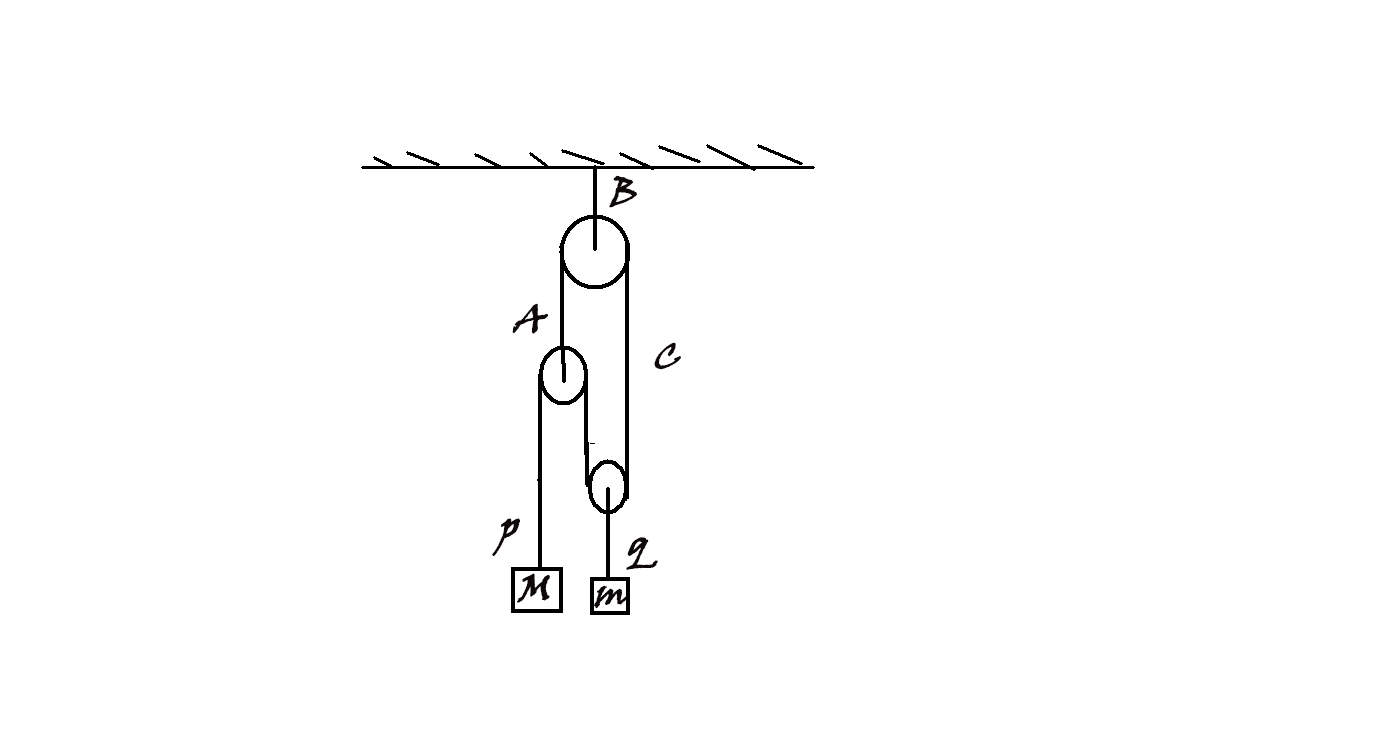# A classical mechanics problem by Pankaj JoshiThe arrangement shown in figure has $2$ masses $M = 4\,\text{kg}$ and $m= 1\,\text{kg}$. The magnitude of acceleration of mass $M$ and $m$ is $p$ and $q$ respectively. The value of $p+q$ is $(g=10 \text{m/s}^2)$ :

×